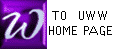NAMING ELEMENTARY ROW OPERATIONS and       PIVOTING

 An elementary row operation is one of the following changes made to the rows of a matrix, as also described in our text (Rolf Pg 89):

 Interchanging two rows

 Multiplying or dividing each element of a row by the same non-zero number "c".


 Adding to a row (the TARGET ROW) a non-zero multiple "c" of another row (the TOOL ROW). While pivoting (see below), the TOOL row is the PIVOT row.

Prof McFarland's Names Row Operation Text's Names
  rm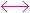rn or rn = Rm and rm = Rn
Interchange
rows rm and rn
 RmRn
  c. rm = Rm or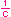. rm = Rm
 MultiplyorDivide by c
c. Rm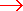Rm
or. RmRm
  rm + c. rn = Rm
of Row n
to Row m
Rm + c. RnRm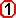3 -12211r2 - 3r1 = R2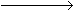10 -1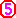25(0.2)r2 = R210 -1121r1 + r2 = R110 0131PIVOTING
 Prof McFarland's names for row operations differ a bit from the names used in our text.

PIVOTING (or formally, a PIVOT TRANSFORMATION) uses row operations to change one matrix entry (the PIVOT) to "1" (see P1 below), and then (see P2 below) to change all other entries in the pivot's column into ZERO's. Also see our text (Rolf Pg 92).

Once a pivot is chosen, the row operations of pivoting MUST BE AS FOLLOWS:

P1
 Change the chosen pivot into "1" by dividing the pivot's row by the pivot number, as was done in the 2nd row operation in the above example.

P2
 Change the remainder of the pivot's COLUMN into 0's by adding to each row a suitable multiple of the PIVOT ROW, as was done in the 1st and 3rd row operations in the example above.

Note 1. The number changing to "1" is called a PIVOT, is usually encircled, and cannot be zero.

Note 2. Completely finish both P1 and P2 (above) before choosing another pivot.

Note 3. Prof McFarland has designed a time-saving interactive PIVOT ENGINE: check it out!

Note 4. (Again) Our brief example of pivoting, with row names color-matched with matrix rows:3 -12211r2 - 3r1 = R210 -125(0.2)r2 = R210 -1121r1 + r2 = R110 0131Thus, in the example just above, there are actually TWO pivot transformations shown. One consists of the 1st row operation alone, whose pivot was the number "1" in the original matrix, encircled; the other consists of the 2nd and 3rd row operations as a pair, whose pivot is the encircled number 5.

 Note 5. Use pivoting when possible during Gauss/Jordan (Rolf, section 2.2) and when you find matrix inverses (Rolf, section 2.6). Though pivoting is not required to obtain correct answers in these sections, pivoting WILL be required later during simplex method, and now is a good time to develop the habits needed at those later times.